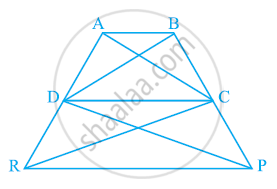Share

# In the given figure, ar (DRC) = ar (DPC) and ar (BDP) = ar (ARC). Show that both the quadrilaterals ABCD and DCPR are trapeziums. - CBSE Class 9 - Mathematics

ConceptTriangles on the Same Base and Between the Same Parallels

#### Question

In the given figure, ar (DRC) = ar (DPC) and ar (BDP) = ar (ARC). Show that both the quadrilaterals ABCD and DCPR are trapeziums.#### Solution

It is given that

Area (ΔDRC) = Area (ΔDPC)

As ΔDRC and ΔDPC lie on the same base DC and have equal areas, therefore, they must lie between the same parallel lines.

∴ DC || RP

Therefore, DCPR is a trapezium.

It is also given that

Area (ΔBDP) = Area (ΔARC)

⇒ Area (BDP) − Area (ΔDPC) = Area (ΔARC) − Area (ΔDRC)

⇒ Area (ΔBDC) = Area (ΔADC)

Since ΔBDC and ΔADC are on the same base CD and have equal areas, they must lie between the same parallel lines.

∴ AB || CD

Therefore, ABCD is a trapezium.

Is there an error in this question or solution?

#### APPEARS IN

NCERT Solution for Mathematics Class 9 (2018 to Current)
Chapter 9: Areas of Parallelograms and Triangles
Ex. 9.30 | Q: 16 | Page no. 164

#### Video TutorialsVIEW ALL 

Solution In the given figure, ar (DRC) = ar (DPC) and ar (BDP) = ar (ARC). Show that both the quadrilaterals ABCD and DCPR are trapeziums. Concept: Triangles on the Same Base and Between the Same Parallels.
S